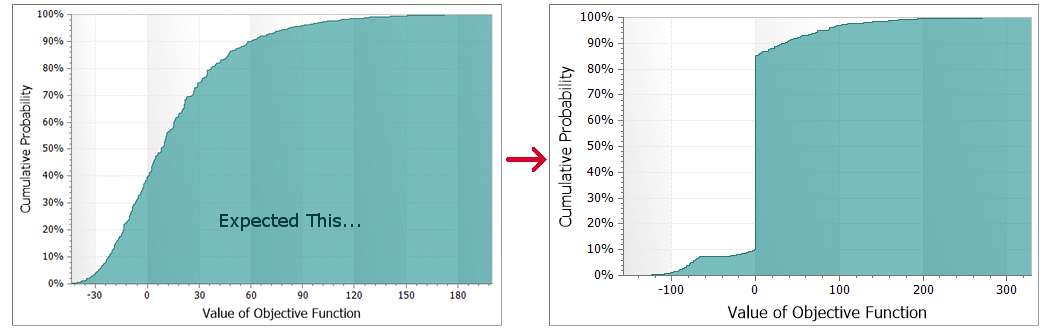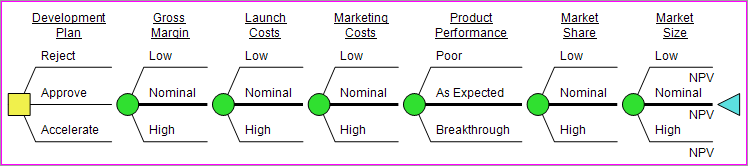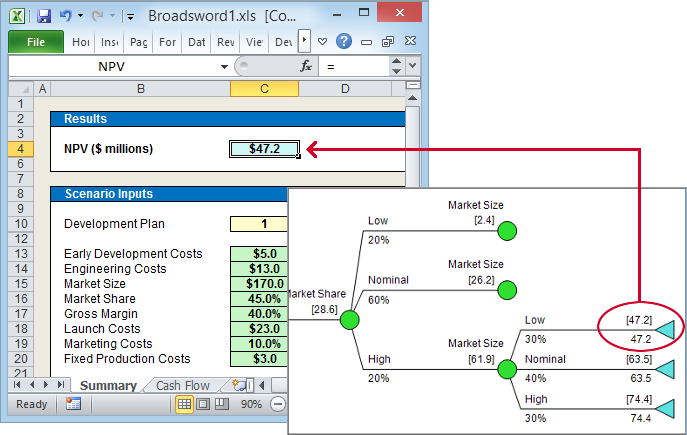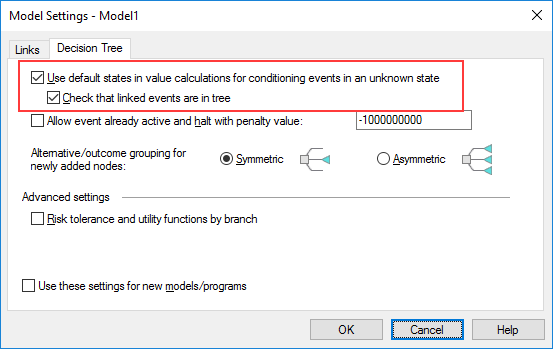# 6 Ways to Validate your DPL/Excel Model

## A list of 6 steps you can take to validate that a DPL/Excel model is computationally correct

This blog posts describes various steps that may be taken to validate that a DPL/Excel model is computationally correct. The actual testing/validation required will depend on the particulars of your model and the circumstances of the analysis. Accordingly, this is more of a menu one can pick and choose from rather than a checklist. If you use other methods not listed, and they may be generally useful, please add them in the comments section below.

One thing to keep in mind – model validation is an exercise in value of information. One can never be 100% certain that a model contains no errors. A model has been validated when the risk of a material (~= decision sensitive) error is acceptable given the stakes of the decision and the cost (\$ and time) of further validation.

### 1. Basic Model Run

It is a good sign if the model runs, but you should also scan the results for:

• unexplained vertical or horizontal lines in the risk profile
• “ties” where decision/chance states all produce the same expected value (usually an indication that something is unhooked)### 2. Test Reasonableness of Scenarios

• Branch control all uncertainties to their base case and run an analysis. Check that the expected values agree with those in the spreadsheet for the decision combinations• In the spreadsheet, change several uncertainties and make sure the value moves in the right direction
• Choose several different scenarios, drill down the policy tree to find the value (NPV) for each one; compare with the spreadsheet for that scenario and inspect the cash flows and other main results in the spreadsheet for each one### 3. Directional Testing

• Control as in 2a then uncontrol one uncertainty at a time and re-run, making sure the results move in the right direction. And then do the reverse – uncontrol all uncertainties and control them one at a time for each run

### 4. Range check Excel model

• Add formulas to the Excel model to verify range conditions (e.g., sales volumes >= 0, percentages between zero and 100%, summing to 100%, mutually exclusive alternatives not both chosen, etc)
• Make the main value return an error if the ranges are violated. For example, the formula for DPL_NPV could be =IF(QACheck=0, NPV, #N/A)

### 5. Ignore Conditioning Unknown State Setting

You may have developed your Excel model such that if certain decision alternatives are selected then the spreadsheet ignores certain input variables. If you then build an asymmetric tree and do not want DPL to check for dependencies based on the influence diagram, you should have the Tree-based dependency checking option turned on.

DPL still sends values for nodes (the Default state for uncertainties) that are skipped in an asymmetric tree. So you must ensure the Excel spreadsheet is coded so that events that are omitted on some of paths of the tree don’t matter on those paths. To verify this

• For each event that is omitted, inspect the spreadsheet formulas and make sure that they logically isolate the input for the omitted event in the scenarios where it is omitted
• Turn off “tree-based dependency checking”. Add branch controlled tree instances to the “short” paths to make the model compile. The added instances should be controlled to a branch other than the default. Make sure the results are the same.### Second Pair of Eyes

Have the other person:

• inspect the Influence Diagram and Decision Tree, including node values and get/pays
• inspect all the formulas in the spreadsheet or at least those sections touched by the DPL switches